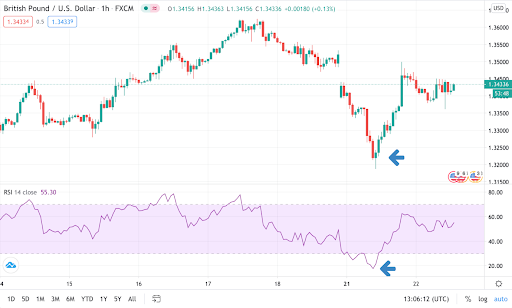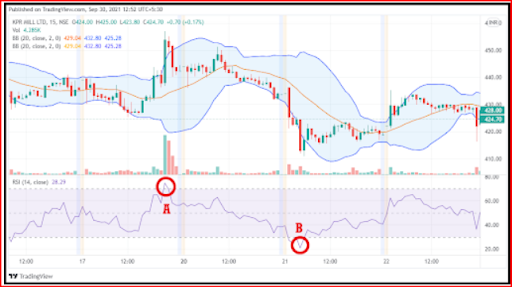## Best Indicators to use with RSI

Relative Strength Index (RSI) is used in technical analysis that measures the change in price movement of a security. Generally, it is used as a momentum indicator independently.

However, if used with other indicators, RSI proves to indicate accurate signals for security. RSI indicators can solely help you to analyse the buy and sell signals, but to grab an accurate indicator, you can use other indicators with RSI.

## 1. RSI with MACD

RSI and moving average convergence divergence (MACD) form a momentum indicator to understand intraday trading with the concept. To understand what is RSI in stock market with macd, let’s move to learn further.

Using MACD with RSI together can help traders spot buying opportunities in a trade. These two are the most popular momentum indicators and thus, when used together can help in validating numerous opportunities.

1.1 RSI displays values which oscillate between 0 and 100. Further, the two important levels that traders analyse are 30 and 70 levels. Suppose the RSI drops below 30 it is oversold and if crosses above 70 it is overbought.

1.2 MACD displays two different moving averages through short and long combinations. For example, the golden cross is a bullish indicator inviting long traders and the death cross is a bearish indicator inviting bearish trades. The downward and upward momentum is analysed through RSI.

We combine MACD and RSI to define the price momentumWhen you combine both indicators you maximise the value you get out of these to plant your trade. It is essential to have your strategy while analysing the combination of these indicators.

If RSI shows momentum in a certain direction, then use MACD to confirm the direction. If the other indicator agrees, then it is wise to take the trade. On the other hand, if MACD and RSI give out split views, then you should wait for another trade opportunity.

MACD indicators use with RSI are considered momentum indicators that are both accurate and reliable. Usually, beginner traders assume that it is best to use a single indicator, however it is always advised to use more than one indicator to confirm a certain tradeUse MACD and RSI to confirm the following:

1.3 Validation of Price Momentum

Price momentum is measured by using RSI which helps in assessing the price levels of stock. RSI measures the price momentum through the differences in time created on the chart.

MACD, on the other hand, shows the relationship of exponential moving averages that confirms the trend-following momentum. In this manner, one indicator verifies the work of another indicator and a trader may find the confidence to enter a trade.

1.4 Exit a Position during Divergence

A trader can exit a position when RSI and MACD indicators confirm the shift in momentum in the stock. A trading strategy describes when to enter and exit the trade.

Using the combination of RSI and MACD traders pick out which indicator is weakening the signal of another. A weak signal is the first sign to exit a position during divergence.

## 2. RSI with Bollinger Bands

The strategy of RSI with Bollinger bands is used to confirm the relative strength of a trending stock. For example, if a stock is in either uptrend or a downtrend, using RSI with Bollinger brands confirms the trend.

To create a strategy with RSI & Bollinger bands, the three components are essential to analyse.

• The Bollinger bands consist of the centre line which is the default line showing 20 days

•  The second line is the upper limit line which is the standard deviation of the centre line with a value of plus 2.

• The third line is the lower limit line which is the standard deviation of the centre line with a value of minus 2.

Bollinger bands and RSI combination suggests that when the price moves in an upward direction and closes beyond the upper limit line of the Bollinger band, the trader should look for a sell signal.

When the price moves downward direction and closes below the lower limit line of the Bollinger band, the trader should observe for a buying signal.For example, the above chart shows two points A and B where at point A the RSI shows an extreme of 75 and it is in the upper limit line of the Bollinger band. It indicates a sell signal.

In point B, the RSI is valued below 30 or around 20 which shows the extreme lower limit value in the Bollinger band. Thus, a buy signal is focused on this case.

## 3. RSI with EMA

Exponential moving averages strategy (EMA) are used in technical analysis, which is a type of moving average to find the significance of the data points. It measures the direction of the trend over some time.

Using EMA with RSI allows a greater weight on the recent analysis of data points. RSI is used with EMA to help traders respond more quickly while taking a trade. The recent price changes are analysed by EMA while the RSI obtains the sign of trend changes.

EMA consists of crossover strategies that consist of slow-moving average line and fast-moving average line.

During crossovers, the EMA line crosses the slow-moving line from below to show a buying signal.

If the EMA line crosses the slow-moving line from above, then it is a sell signal.

The buy and sell strategies through EMA are simple as it sounds. Although, there is a high chance of the trend becoming difficult or fake in the market. When the cross-over fails to take place, the EMA line is unable to depict the correct signals.

In this situation, a trader should consider using RSI by analysing the trend of the market through the 30 and 70 levels. RSI is used with EMA to understand the oversold and overbought areas. A trader should be able to confirm EMA analysis when RSI confirms a valuable trend.

A trader should consider using this strategy when considering buying or selling over the correct signals. Remember that when the EMA crossover happens with a single point, the trader should consider entering as per the RSI below 30 or above 70.

Other than EMA, a trader can also use RSI with Simple Moving Average (SMA) which requires a related timeframe to trade in the market.## Conclusion

We hope you found these three strategies useful to learn and make sure to practice them in the combination suggested. One should always remember that values for traders increase when they understand their own practised strategies. The type of stock to trade, trading time frames to use and the indicators to implement the technical analysis.

Apart from the other three, more indicators can be used with RSI such as the Average Directional Index which we will introduce in coming blogs. Make sure to read the information and keep learning.

Key Takeaways

• RSI values oscillate between 0 and 100.

• RSI is best combined with MACD, Bollinger Bands and EMA

• MACD and RSI help in validating price momentum and exit position strategies

• RSI with Bollinger bands is used to confirm the relative strength of Trends

• RSI with EMA measures the direction of the trend and finds signals

• Bollinger bands consist of a centre line, upper limit line and lower limit line

• MACD displays a bearish and bullish trend through the golden cross and death cross.

• EMA crossing a slow-moving line from above causes a selling signal and crossing a slow-moving line from below showing buying signal.